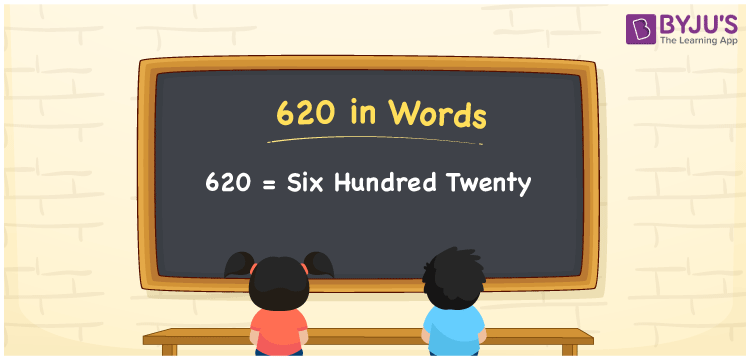# 620 in Words

620 in words is written as Six hundred twenty. In both the International System of Numerals and the Indian System of Numerals, 620 is written as Six hundred twenty. The number 620 is a Cardinal Number as it represents some quantity. For example, “620 applications were received”.

 620 in Words Six hundred twenty Six hundred twenty in Number 620

## 620 in English Words

We write 620 in English Words using the letters of English alphabets. Therefore, we read 620 in English as “Six hundred twenty”## How to Write 620 in Words?

To write 620 in words, we shall use the place value chart. In the place value chart, write 6 in the hundreds, 2 in the tens, and 0 in the ones, respectively. Now let us make a place value chart to write the number 620 in words.

 Hundreds Tens Ones 6 2 0

Thus, we can write the expanded form as

6 × Hundred + 2 × Ten + 0 × One

= 6 × 100 + 2 × 10 + 0 × 1

= 600 + 20 + 0

= 620

= Six hundred twenty.

620 is a natural number, the successor of 619 and the predecessor of 621.

620 in words – Six hundred twenty

• Is 620 an odd number? – No
• Is 620 an even number? – Yes
• Is 620 a perfect square number? – No
• Is 620 a perfect cube number? – No
• Is 620 a prime number? – No
• Is 620 a composite number? – Yes

## Frequently Asked Questions on 620 in Words

Q1

### How to write 620 in words?

620 in words is written as Six hundred twenty.
Q2

### How to write 620 in words in the International and Indian System of Numerals?

In both the system of numerals, 620 is written as Six hundred twenty.
Q3

### What are the factors of 620?

The factors of 620 are 1, 2, 4, 5, 10, 20, 31, 62, 124, 155, 310 and 620.